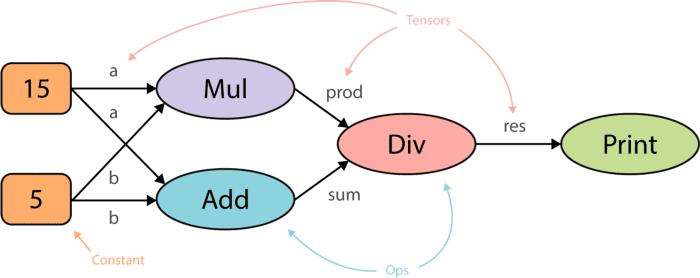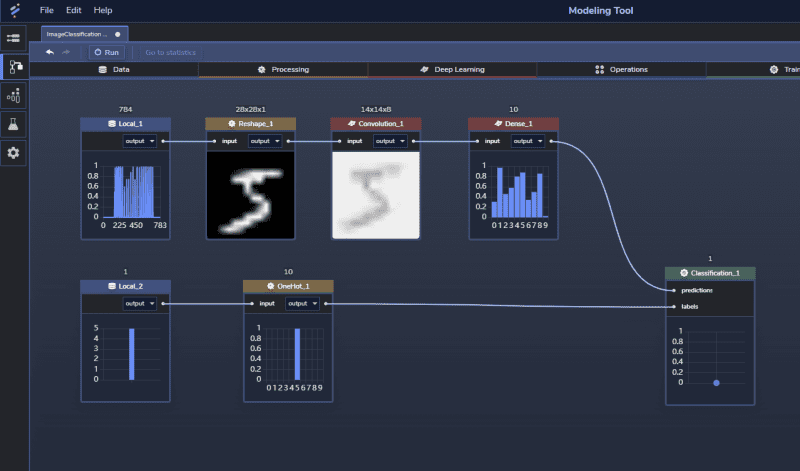Topics: AI | Data Science | Data Visualization | Deep Learning | Machine Learning | NLP | Python | R | Statistics

KDnuggets Home » News » 2020 » Nov » News, Education » Primer on TensorFlow and how PerceptiLabs Makes it Easier ( 20:n44 )

# Primer on TensorFlow and how PerceptiLabs Makes it Easier

With PerceptiLabs, beginners can get started building a model more quickly, and those with more experience can still dive into the code. Given that PerceptiLabs runs TensorFlow behind the scenes, we thought we'd walk through the framework so you can understand its basics, and how it is utilized by PerceptiLabs.

By Martin Isaksson, Co-Founder and CEO of PerceptiLabs

In A New Visual Approach to Machine Learning Modeling, we talked about how TensorFlow is one of the most popular machine learning (ML) framework today, but it’s not necessarily an easy one for beginners to start building ML models.

That's why we decided to create a GUI on top of TensorFlow. With PerceptiLabs, beginners can get started building a model more quickly, and those with more experience can still dive into the code. Both types of users benefit from PerceptiLabs' rich set of visualizations that include the ability to see a model's architecture, experiment and see how parameter and code changes affect models in real time, and view a rich set of training and validation stats.

Given that PerceptiLabs runs TensorFlow behind the scenes, we thought we'd walk through the framework so you can understand its basics, and how it is utilized by PerceptiLabs.

### Models as Graphs

The first thing you need to know is that in TensorFlow, ML models are represented as data flow graphs (aka computational graphs). In fact, the name TensorFlow is derived from this graph-based design where tensors are represented as edges which flow through the graph's nodes, and operations are encapsulated in the nodes themselves. Figure 1 shows a very basic example of a data flow graph for performing mathematical operations:Figure 1: Example of a basic data flow graph for mathematical operations. Source

Here, the input consists of scalars, which flow as tensors into the first set of operations (multiplication and addition). The output of those operations then flows as tensors into the division operation and the subsequent value into a print operation.

Representing an ML model in a data flow graph means that TensorFlow can optimize its execution such as performing operations (e.g., multiplication and addition in the example above) in parallel.

The next part to understand is the TensorFlow Distributed Execution Engine which is the underlying technology that constructs, optimizes, and runs these graphs. This code library performs all of the logic and as the name suggests, can distribute operations across a variety of hardware including CPUs, TPUs, and GPUs (for hardware accelerated execution), OS's, and even across servers.

Programmers use the TensorFlow Distribution Execution Engine via TensorFlow's APIs. These APIs allow programmers to build, configure, and run their models in a variety of computer languages. For TensorFlow, its Python implementation is arguably the most popular in the ML world. Thus TensorFlow's Python Frontend is the name of the entity through which TensorFlow's APIs are exposed. TensorFlow is composed of "layers" of APIs where the different layers abstract ML concepts to varying degrees:Figure 2: Summary of TensorFlow's architecture and API layers. Source

Starting near the bottom of the stack, the following APIs are available:

• Layers: the most raw level of abstraction for building graphs of operations and tensors via explicit constructs. While TensorFlow 1.0 started with this layer, it has since been removed in TensorFlow 2.0.
• Datasets: APIs to load and manipulate data (e.g., tf.data.Dataset can be used to load training data, shape it, and iterate on its output). This API can be used in conjunction with other API layers.
• Estimator: high-level APIs to train, evaluate accuracy, and perform inference on a model. Programmers can customize estimators using the tf.estimator.Estimator object.
• Keras Model: a high-level API that provides the highest level of abstractions allowing ML practitioners to focus on building neural networks, without having to deal with low-level constructs and computations (e.g., the underlying algorithms and math). Keras originated outside of TensorFlow but was eventually implemented on top of TensorFlow's lower-level APIs.
• Pre-made (aka Canned) Estimators: pre-defined Estimators included with TensorFlow for common types of ML models and problems (e.g., tf.estimator.LinearRegressor for linear regression).

TensorFlow programs are compiled by the language-specific front end using TensorFlow's Session object. This object can perform a number of operations including analyzing a model's graph and running its operations in parallel when possible. Figure 3 illustrates how the model's graph is passed through the Session object for execution on the underlying hardware:Figure 3: Summary of how a model's tensors (edges) and operations (nodes) are passed to TensorFlow's session object for execution across hardware. Source: PerceptiLabs

### Output Formats

Normally programmers work with a TensorFlow API via language-specific source code files (e.g., .py files for Python) and then compile or execute those programs (or scripts in the case of Python). In using the API to train the model, developers have the option to save checkpoints which serialize the values of the model's parameters at certain points. This allows programmers to resume training at a later point and to share weights when collaborating with others.

When the script exports the fully trained model, it can write it to the following two file formats:

• SavedModel Format (.pb files): Protobuf file format that includes all aspects of the model including its execution graph, custom objects, and layers. This is generally the preferred and most portable export format and is the format that PerceptiLabs exports to.
• HDF5 (.h5 files): a format that originated with Keras which saves the model’s architecture but requires custom code to load custom objects for inference.

### A GUI and Visual API for TensorFlow

Perhaps the biggest challenge in using TensorFlow directly for both beginners and advanced users, is in trying to visualize the model. Not only is it difficult to visualize TensorFlow's graph representation, but imagine trying to visualize all those underlying tensor connections which can number in the millions? Then, imagine trying to visualize how changes to the hyperparameters and code affect that model, a problem that is further compounded as the model becomes bigger and more complex.

That's why we see PerceptiLabs as both a GUI and visual API for TensorFlow. The GUI aspect comes from PerceptiLabs’ components, which implement TensorFlow code behind the scenes. Some components correspond directly to the traditional notion of ML model "layers", while others are more general. Furthermore, the layer components allow users to focus on how those layers relate to other elements of the model, rather than trying to visualize graphs of tensors and connections.

The visual API concept is based on the concept of dropping components into a model, where each component wraps and abstracts away the underlying code implementation, similar to how a high-level API like Keras wraps many operations into one-liners of code. Similarly, we've wrapped many deep learning operations into their own components so that you don't need to think about those millions of neural connections, but can instead, focus on the organization and logic of your model from a higher level.

For example, remember that Session object we mentioned above? PerceptiLabs' Classification component pulls together everything in your model to perform classification. The component takes predictions and labels as input which you provide from other components by simply connecting them. Then, when it comes time for training, the Classification component, instantiates a Session object and invokes a number of its methods to run a classification algorithm. Of course, you needn't worry about writing all of this code, as the component has done this for you, but programmers are more than welcome to view and modify that code. Of course, all users, programmers or not, can easily adjust that component's hyperparameters through a few simple clicks.

An important aspect that enables all of this visualization is the visual previews of each component. PerceptiLabs' model editor is able to show these because it uses the first instance/sample of data from the underlying data source exposed by each Data component in a model. After the connections between components are made, subsequent changes to any component's settings or underlying TensorFlow code cause each preview in the model to be updated on the fly.Figure 4: Screenshot of a simple image classification model in PerceptiLabs, and the previews displayed for each component driven by the image and label data provided to the model.

For example, in Figure 4 the first data component contains images of handwritten digits, while the second data component contains the corresponding labels. PerceptiLabs' Data component automatically extracts the first data element from its underlying data source (first image and first label in the example above), and it's this data that ultimately flows through the model to display previews of the components' transformations. Then during training, you can click on any component to see how it transforms each data element as TensorFlow iterates over the data set(s).

### Get Started Today!

TensorFlow is a popular and powerful ML framework, but makes models difficult to visualize and ultimately needs technical users to implement solutions.

We hope that this blog has provided you with the fundamentals of how TensorFlow works and how, with PerceptiLabs, you can more easily build, train, and optimize your ML models.

If you haven't tried our free version of PerceptiLabs yet, follow our Quickstart Guide or enter the following in a command-line window:

```\$ pip install perceptilabs \$ perceptilabs```

And be sure to register when prompted by the app!

Top Stories Past 30 Days
Most SharedGet KDnuggets, a leading newsletter on AI, Data Science, and Machine Learning